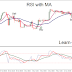## Top Ad unit 728 × 90

RSI removes volatility and gives signals flowing along with moving averages.
This tool for trading is constructed as follows:
2 moving averages on the price (7 simple and weighted 34) and two moving averages on the indicaatore RSI (4 smooted 21 and weighted).

Trend up
The 7 period Ma on price si above of the 34 Ma on price and
the 4 period MA on RSI is above of the 21 period on RSI.

Trend is down
The 7 period Ma on price si below of the 34 Ma on price and
the 4 period MA on RSI is below of the 21 period on RSI.

Trend is sideways up
The 7 period Ma on price si above of the 34 Ma on price and
the 4 period MA on RSI is below of the 21 period on RSI.

Trend is sideways down
The 7 period Ma on price si below of the 34 Ma on price and
the 4 period MA on RSI is below of the 21 period on RSI.

Time Frame 4H or Higher.

Another way of interpreting this technique and to add to the Levels of 60 and 40.
The area between these two levels is defined: the lateral area.
Sell below the level 40 of the IHR.
If RSI is between 40 and 60 not to trade.

Example trend up
The 7 period Ma on price si above of the 34 Ma on price and
the 4 period MA on RSI is above of the 21 period on RSI.
RSI above 60 level.

Example trend down
Trend is down
The 7 period Ma on price si below of the 34 Ma on price and
the 4 period MA on RSI is below of the 21 period on RSI.
RSI below 40 level.

RSI with Moving Averages tool for tradingReviewed by learn forex trading on December 05, 2015 Rating: 5